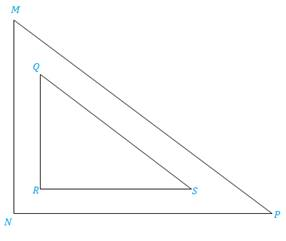Chapter 5.3, Problem 21E### Elementary Geometry for College St...

6th Edition
Daniel C. Alexander + 1 other
ISBN: 9781285195698

#### Solutions

Chapter
Section### Elementary Geometry for College St...

6th Edition
Daniel C. Alexander + 1 other
ISBN: 9781285195698
Textbook Problem
1 views

# In Exercises 17 to 24, complete each proof.Given: R Q M N = R S N P = Q S M P Prove: ∠ N ≅ ∠ RPROOF Statements Reasons 1. ?2. ?3. ? 1. Given2. S S S ∼ 3. CASTC

To determine

To prove:

The statement NR if the proportions are given RQMN=RSNP=QSMP.

Explanation

Definition:

SSS :

If three sides of one triangle are proportional (in length) to three corresponding sides of a second triangle, then the triangles are similar.

CASTC:

Corresponding angles of similar triangles are congruent.

Description:

Given that RQMN=RSNP=QSMP.

The given figure is shown below.

Figure

From the given figure, it is observed that RQMN=RSNP=QSMP are three sides of a triangle QRS are proportional to three corresponding sides of a triangle MNP.

The above mentioned SSS definition, the two triangles MNP and QRS are similar since the three sides of one triangle are proportional to three corresponding sides of a second triangle. Hence, ΔMNPΔQRS

### Still sussing out bartleby?

Check out a sample textbook solution.

See a sample solution

#### The Solution to Your Study Problems

Bartleby provides explanations to thousands of textbook problems written by our experts, many with advanced degrees!

Get Started